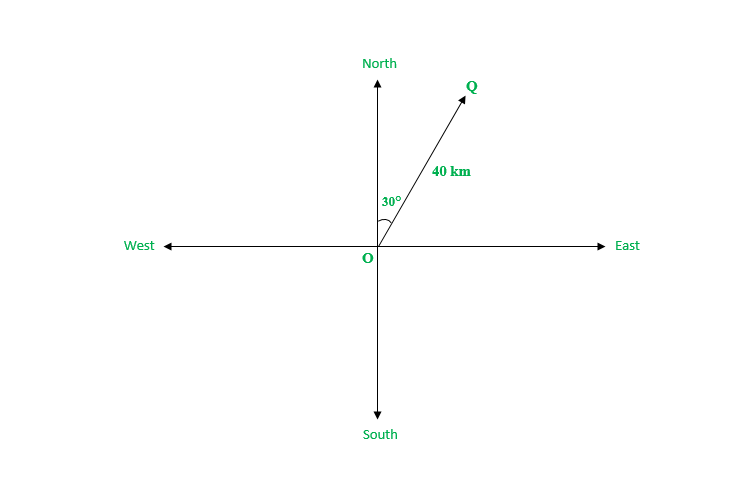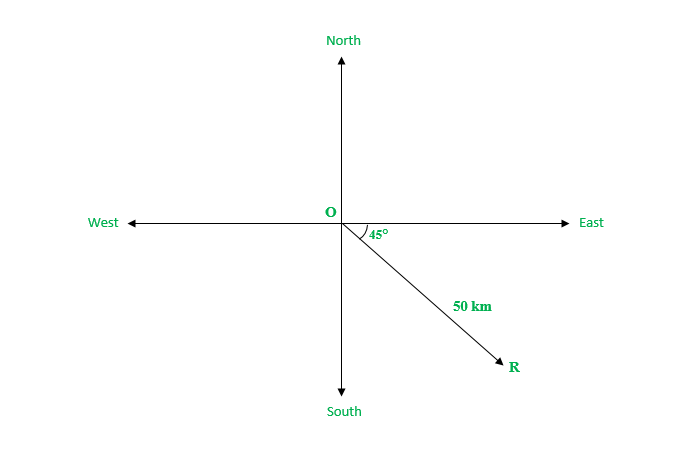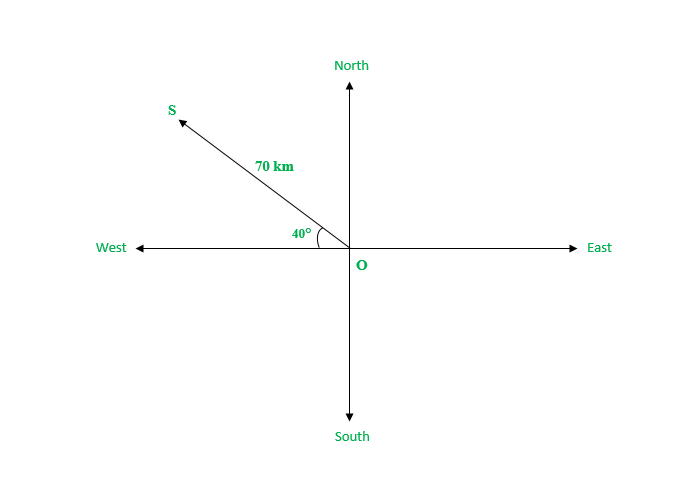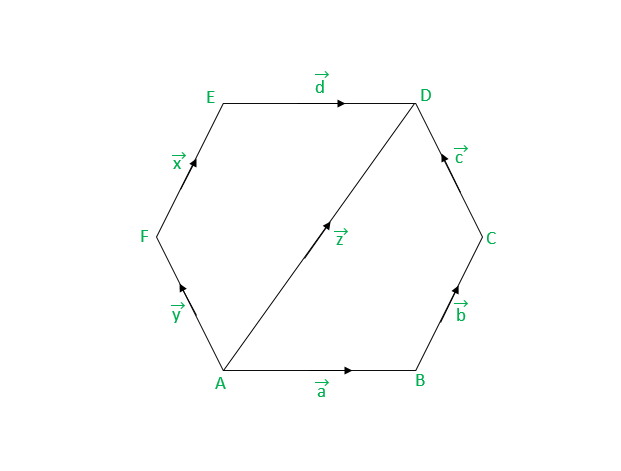# Class 12 RD Sharma Solutions- Chapter 23 Algebra of Vectors – Exercise 23.1

• Last Updated : 03 Jan, 2021

### Question 1. Represent the following graphically:

(i) a displacement of 40 km, 30° east of north

(ii) a displacement of 50 km, south-east

(iii) a displacement of 70 km, 40° north of west.

Solution:

(i) The vector OQ represents the displacement of 40 km, 30° east of north(ii) The vector OR represents the displacement of 50 km, south-east(iii) The vector OQ represents the displacement of 70 km, 40° north of west.### (i) 15 kg       (ii) 20 kg weight       (iii) 45°       (iv) 10 meters south-east       (v) 50 m/sec 2

Solution:

Note: Scalar quantities have only magnitude and are not related to any fixed direction in space. Vector quantities have both magnitude and direction

(i) 15 kg involves only mass – Scalar

(ii) 20 kg weight kg involves both magnitude and direction – Vector

(iii) 45° involves only magnitude – Scalar

(iv) 10 meters south-east involves direction – Vector

(v) 50 m/sec 2 involves magnitude of acceleration – Scalar

### (i) Time period       (ii) Distance       (iii) Displacement       (iv) Force       (v) Work       (vi) Velocity       (vii) Acceleration

Solution:

Note: Scalar quantities have only magnitude and are not related to any fixed direction in space. Vector quantities have both magnitude and direction

(i) Time period involves only magnitude – Scalar

(ii) Distance involves only magnitude – Scalar

(iii) Displacement involves both magnitude and direction – Vector

(iv) Force involves both magnitude and direction- Vector

(v) Work done involves only magnitude – Scalar

(vi) Velocity involves both magnitude and direction – Vector

(vii) Acceleration involves both magnitude and direction – Vector

### (i) Collinear       (ii) Equal       (iii) Coinitial       (iv) Collinear but not equalSolution:

In the given figure Vectors:

(i) a, d ;

b, x, z ;

c,y

are Collinear vectors as they have same or parallel supports

(ii) b, x ;

a, d ;

c, y

are equal vectors as they have same length, the same or parallel support and, the same sense

(iii) a, y, z

are Co-initial Vectors having same initial point

(iv) b, z ;

x, z

are Collinear Vectors but not equal as they are parallel, but their lengths are not the same.

### Question 5. Answer the following as true or false:

(i) a and b vectors are collinear

(ii) Two collinear vectors are always equal in magnitude

(iii) Zero Vector is unique.

(iv) Two vectors having same magnitude are collinear

(v) two collinear vectors having the same magnitude are equal

(i) True. As vectors having same or parallel supports are Collinear

(ii) False. Two Collinear vectors must have same supports and may not be equal in magnitude

(iii) False. Zero vector may not be unique

(iv) False. Two vectors having same magnitude may not be collinear

(v) False. Two collinear vectors having same magnitude may not be equal

My Personal Notes arrow_drop_up